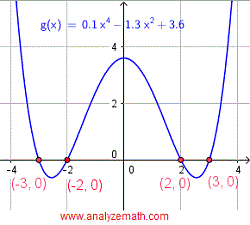# Solve Equations of the Quadratic Form

How to solve equations that may be reduced to quadratic equations using substitution? Questions with detailed solutions are presented. Graphical solutions to these equations are also presented.

Free Practice for SAT, ACT
and Compass Maths tests

## Question 1

Solve the equation 0.1 x4 - 1.3 x2 + 3.6 = 0 .

## solution

Let u = x2 which gives u2 = x4 and rewrite the given equation in terms of u
0.1 u2 - 1.3 u + 3.6 = 0
Solve the above quadratic equation to find u.
u = 4 and u = 9
We now use the substitution u = x2 to solve for x.
u = 4 = x2 gives two solutions: x = - 2 and x = 2
u = 9 = x2 gives two solutions: x = - 3 and x = 3
The four x intercepts of the graph of y = 0.1 x4 - 1.3 x2 + 3.6 are the graphical solutions to the equation as shown below..

## Question 2

Solve the equation: √x = 3 - (1 / 4)x.

## solution

Let u = √x which gives u2= x and rewrite the given equation in terms of u
u = 3 - u 2 / 4
Multiply all terms by 4, simplify and write the above quadratic in standard form and solve it for u.
u 2 + 4 u - 12 = 0
Two solutions: u = - 6 and u = 2
Use the substitution used above u = √x to solve for x.
u = - 6 = √x has no solution
u = 2 = √x has solution x = 4
Below is shown the graph of the right side of the given equation when written with its right side equal to zero. The x intercept of the graph is the graphical solution to the equation as shown below..

## Question 3

Solve the equation: $(3 - \dfrac{4}{x})^2 - 6 (3 - \dfrac{4}{x}) = 16$

## solution

Let y = 3 - 4 / x which gives y2= (3 - 4 / x)2 and rewrite the given equation in terms of y.
y 2 - 6 y = 16
Solve the above equation.
y 2 - 6 y - 16 = 0
y = - 2 and y = 8
y = - 2 and y = 8
Solve for x.
First solution: y = 3 - 4 / x = -2 gives x = 4 / 5
First solution: y = 3 - 4 / x = 8 gives x = - 4 / 5
The graph of the right side of the given equation written with its right side equal to zero. The x intercepts of the graph are the graphical solutions to the equation as shown below..

## Question 4

Solve the equation: 2(x - 1)2 / 3 + 3(x - 1)1 / 3 - 2 = 0.

## solution

Let y = (x - 1)1 / 3 which gives y2= (x - 1)2 / 3 and rewrite the given equation in terms of y.
2 y 2 + 3 y - 2 = 0
y = - 2 and y = 1 / 2
Solve for x.
y = (x - 1)1 / 3 = - 2 gives x = -7
y = (x - 1)1 / 3 = 1 / 2 gives x = 9 / 8
The graph of the right side of the given equation is shown below and its x intercepts are the graphical solutions to the given equation..

## Question 5

Find all real solutions for the following equation: $2\left(\dfrac{2}{x-3}\right)^2 -\dfrac{2}{x-3} - 3 = 0$

## solution

Let u = 2 / (x - 3) which gives y2 = (2 / (x - 3))2 and rewrite the given equation in terms of u.
2 u 2 - u - 3 = 0
Solve for u.
u = - 1 and u = 3 / 2
Solve for x.
y = 2 / (x - 3) = - 1 gives x = 1
y = 2 / (x - 3) = 3 / 2 gives x = 13 / 3
Below is shown the graph of the right side of the equation and its x intercepts which are the graphical solution to the given equation..